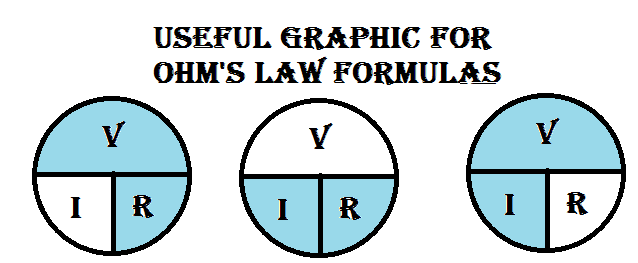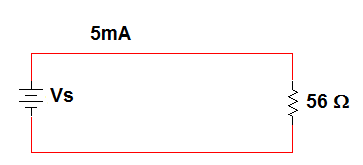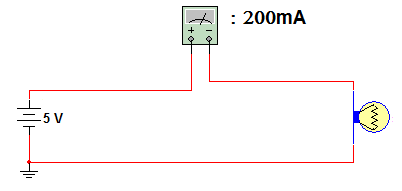Home / Basic Electrical / Ohms Law and Power in Electrical Circuits

# Ohms Law and Power in Electrical Circuits

Want create site? Find Free WordPress Themes and plugins.

This module will enable readers to use Ohm’s Law and electric power formulas to determine the voltage, current, resistance and power in a basic DC circuit.

Objective

Learner will be able to:

• Use Ohm’s Law to determine voltage, current, or resistance
• Define Energy and Power
• Calculate Power in a basic DC circuit.

Orienting Questions

• How do voltage, current and resistance relate?
• How do you describe the power in electric circuits?
• How do you calculate the electric power?

Introduction

Ohm’s Law is a mathematical method for describing the relationship between voltage, current, and resistance. We use this law to find any three of these quantities. With these quantities, we can calculate the power of a circuit as well.

## How do voltage, current and resistance relate?

Ohm’s Law state that current is proportional to voltage and inversely proportional to resistance. The following equation is derived from this law:

$I=~\frac{V}{R}$Given a constant Resistance, when the voltage increases or decreases, so does the current.Given a constant voltage, if the resistance increases, then the current decreases. If the resistance decreases, the current increases.

Example 1

If we were to change the voltage from 5 V to 20 V in a circuit with 100 Ω, we can prove the previous statements.

Circuit with 5 V:

$\text{I}=5\text{ }\!\!~\!\!\text{ V}/100\text{ }\!\!~\!\!\text{ }\Omega =50\text{ }\!\!~\!\!\text{ mA}$

Circuit with 20 V:

$I=20\text{ }\!\!~\!\!\text{ V}/100\text{ }\!\!~\!\!\text{ }\Omega =200~mA$

If we wanted to find the voltage, we can take both sides of the equation and multiply by R, giving us:

V = IR

If we needed to find the resistance, we can divide both sides by I. This gives the equation:

R = V / I

The following graphic (Figure 1) is a good aid for Ohm’s law formulas:I = V/R V = IR R = V / I

Figure 1: Ohm’s Law

Example 2

Find the voltage in the circuit when R = 1M ohms and I = 2A

V = IR

V = (2A) (1Mohms)

V = 2 MV

Find the resistance of a circuit with 25 V and a current of 2mA.

R = (25 V) / (2 mA)

R = 12.5 k ohms

Find the current if a circuit’s supply voltage is 15 V and its resistance is 4700 ohms.

I = V/R

I = 15V / 4700 ohms=3.2mA

## Voltage and Current Linear Relationship

Voltage and current, when resistance is constant, in a resistive circuit are linearly proportional. Linear is when one element is changed by a certain percentage, so is the other. If we increase voltage by 25% then the current will also increase by 25%.

Example 3

Let’s show an example of a circuit with a voltage change by 50%. We will find the current and show that it changes by the same percentage.

Using the following circuit:Figure 2

I = V / R

I = 10V / 100 Ω = 100 mA

Now let’s increase the voltage by 50%

10V (50%) = 5V

10V +5V = 15V

I = 15V / 100 Ω = 150 mA

Did the current increase by 50%? Let’s check.

100mA (50%) = 50mA

100mA + 50mA = 150mA

## Applying Ohm’s Law

In this section there are some examples of applying ohms law to find the three quantities of a circuit. Ohm’s Law is a very simple tool for analyzing electrical circuits. It relates to the electrical quantities voltage, current and resistance to each other.

OHM’s law states:

Current is directly proportional to voltage

Current is inversely proportional to resistance

## Ohm’s Law Current Calculation

Find the current in the following circuit shown in Figure 3:Figure 3

I = V/R

= 10V / 1kΩ

= 10mA

Not always will the numbers be whole numbers. More times than not, you will encounter numbers that are not whole numbers.

Example 4

Given a voltage of 25 V with a resistance of 5.2 k ohms, what is the current through the circuit?

I = V/R

= 25V / 5.2 k ohms

= 4.8 mA

## Ohm’s Law Voltage Calculation

Given I = 5 mA in the circuit shown in Figure 4, Calculate the voltage?Figure 4

V = IR

= (5mA)(56Ω)

= 280 mV

Example 5Figure 5

In the circuit shown in Figure 5, if the resistance is 200 ohms and the current is 2 mA, find the voltage.

V= RI

V = (200) (.002)

V =0.4 V

## Ohm’s Law Resistance Calculation

Find the resistance of the light bulb in the circuit shown in Figure 6:Figure 6

R = V / I

= 5V / 200mA

= 25 Ω

## Power in Electric Circuits

As in a light bulb, when there is current through a resistance there is a form of energy from heat, called thermal energy, which is given off from the bulb. Components in an electrical circuit must be able to dissipate an amount of energy in given amount of time.

In this section, we will define power and energy and learn the calculations involved with them.

Energy (W) is defined as the ability to do work. Power (P) is the rate that energy is used.

P = W / t

If you wanted to find the energy you could multiply the power by time:

W = P. t

The SI unit for energy is the joule (J). The unit for power is called a watt and is symbolized by W. Note that the W for work and watt are the same. Do not get them confused.

Example 6

What is the power when the amount of energy is 200J used in 5 seconds?

P = W / t

= 200J / 5s

= 40W

Even though the joule has been defined as a unit of energy, there is another term to express it. The kilowatt-hour (kWh) is a more common unit for energy since most electrical companies deal with huge amounts of energy. A kilowatt-hour is equal to 1000 W of power for 1 h.

Example 7

If we had a light bulb that was 100 W and it burned for 10 hours, it would use 1 kWh of energy.

W = P. t = (100W)(10h) = 1000 Wh = 1kWh

Current through a resistance causes collisions of the electrons. These electrons heat up and pass the heat through the resistance. This causes a conversion of electrical energy to thermal energy, as in Figure 8 below.Figure 8

Power in an electrical circuit depends on the amount of the resistance and the amount of current:

P = I2R

There are two more equations derived from this equation by substitution:

P = VI

P = V2 / R

These three equations are known as Watt’s law.

Example 8

Let’s calculate the power in the next few examples:

Given 500Ω and current of 2 A:

P = I2R

= (22)(500)

= 2000 W = 2kW

Given 10 V and 2 A current:

P = VI

= (10) (2)

= 20 W

Given 25 V and 20 Ω resistance:

P = V2 / R

= (25)2 / 20

= 625 / 20 = 31.25 W

Resistors have a power rating, which is the maximum amount of power they can dissipate before they become damaged by the heat buildup.

Power ratings come in a variety. Metal-film resistors range from 1/8 W to 1 W. It is important to remember that the power dissipated by a resistor should be lower than the power rating. If a resistor dissipates .25 W of power then a ½ W rating must be used.

Power supply efficiency is the ratio of output power (POUT) to the input power (PIN).

Efficiency = POUT / PIN

Since input power is always more than the output power because the internal circuit to operate the power supply, there is a power loss. The following equation is used to calculate the power loss.

PLOSS = PIN – POUT

Example 9

A power supply requires 20 W of input power. It produces an output power of 15 W. What is the efficiency and the power loss of the power supply?

Efficiency = POUT / PIN

= 15 W / 20 W

= 75%

PLOSS = PIN – POUT

= 20 W – 15 W

= 5 W

Summary

• In an electrical circuit, the voltage and current are proportional. The current and resistance are inversely proportional.
• Power is the measurement of energy. Energy occurs anytime work is performed.
• We can use Watt’s Law to calculate power in an electrical circuit using current, voltage, and resistance.

True or False: Read the following questions and determine whether the statement is true or false.

1. T F If the current is constant, voltage and resistance are directly proportional.
2. T F If a 47 kΩ resistor carries 5 mA, it’s safe to use a 1W resistor.
3. T F If a circuit’s supply voltage is 15 V and its resistance is 4700 ohms, its current is 3.19 mA.
4. T F If I = 32 mA and R = 469 Ω in a circuit, the voltage would equal 12 V.
5. T F When V = 50 V and I = 37 mA the power dissipated by the resistor would be 1.85 W.

Multiple Choice: Read the following questions or statements and select the best answer.

6. If a resistor is rated at ½ W, it:

1. Can safely dissipate ½ W of power
2. Always dissipates ½ W
3. Always provides ½ W of power
4. Can only dissipate more than ½ W of power

7. Power, in electric circuits, can best be defined as:

1. The rate of motion is exerted over time
2. The rate current flows over time
3. The rate voltage is applied over time
4. The rate energy is used over time

8. If V = 100 V and I = 1 mA, the power dissipated by the resistor equals:

1. 10 W
2. 1 W
3. 100 mW
4. 10 mW

9. If V = 25 V and R = 50 kΩ, the current equals:

1. 50 mA
2. 5 mA
3. 0.5 mA
4. 2 mA

10. If I = 27 mA and R = 4.7 Ω, the voltage equals:

1. 127 mV
2. 5.74 V
3. 174 mV
4. 7.8 V

11. What is the resistance of a 150 W bulb when it is operating in a circuit with a supply of 125 V?

1. 75 Ω
2. 104 Ω
3. 9375 Ω
4. 2.14 Ω

12. Resistance and current are __________.

1. Directly proportional
2. Inversely proportional
3. Not related
4. Similar to voltage

True OR False

1. T
2. F
3. T
4. F
5. T

MULTIPLE CHOICE

6. A

7. D

8. C

9. C

10. A

11. B

12. B

Did you find apk for android? You can find new Free Android Games and apps.